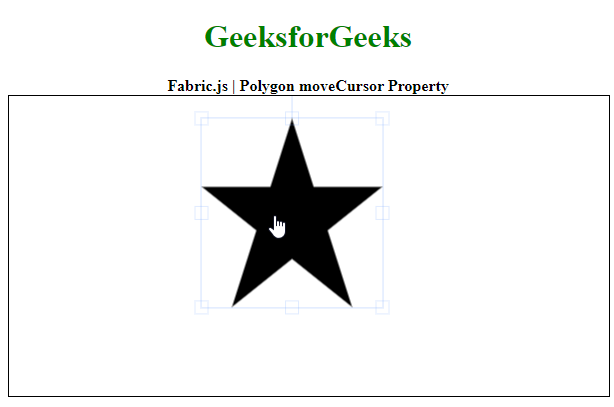Related Articles

# Fabric.js Polygon moveCursor Property

• Last Updated : 31 Aug, 2020

In this article, we are going to see how to set the moveCursor property of a canvas polygon using FabricJS. The canvas polygon means the polygon is movable and can be stretched according to requirement. Further, the polygon can be customized when it comes to initial stroke color, shape, fill color, or stroke width.

To make it possible we are going to use a JavaScript library called FabricJS. After importing the library, we will create a canvas block in the body tag that will contain the polygon. After this, we will initialize instances of Canvas and polygon provided by FabricJS and set the move cursor value of canvas polygon using moveCursor property and render the polygon on the Canvas as given in the below example.

Syntax:

```fabric.Polygon([
{ x: pixel, y: pixel },
{ x: pixel, y: pixel },
{ x: pixel, y: pixel},
{ x: pixel, y: pixel},
{ x: pixel, y: pixel }],
{
moveCursor: string
}
)
```

Parameters: This function accepts a single parameter as mentioned above and described below:

• moveCursor: It is a string that specifies the cursor to be used when moving the canvas object.

Note: The dimension pixels are a must for creating a polygon.

Below example illustrates the Polygon moveCursor property in JavaScript:

Example:

## HTML

 ```<``html``>`` ` `<``head``>``    ````    ``<``script` `src``=``"https://cdnjs.cloudflare.com/ajax/libs/fabric.js/3.6.2/fabric.min.js"``>``    `````` ` `<``body``>``    ``<``div` `style="text-align: center;``              ``width: 600px;">``        ``<``h1` `style``=``"color: green;"``>``            ``GeeksforGeeks``        ````        ``<``b``>``            ``Fabric.js | Polygon moveCursor Property``        ````    ```` ` `    ``<``canvas` `id``=``"canvas"` `width``=``"600"` `height``=``"300"` `        ``style``=``"border:1px solid #000000;"``>``    ```` ` `    ``<``script``>`` ` `        ``// Initiate a Canvas instance ``        ``var canvas = new fabric.Canvas("canvas");`` ` `        ``// Initiate a polygon instance ``        ``var polygon = new fabric.Polygon([``            ``{ x: 295, y: 10 },``            ``{ x: 235, y: 198 },``            ``{ x: 385, y: 78 },``            ``{ x: 205, y: 78 },``            ``{ x: 355, y: 198 }], {`` ` `            ``// Set the cursor``            ``// to a pointer``            ``moveCursor: 'pointer'`` ` `        ``});`` ` `        ``// Render the polygon in canvas ``        ``canvas.add(polygon); ``    `````` ` ``

Output:My Personal Notes arrow_drop_up